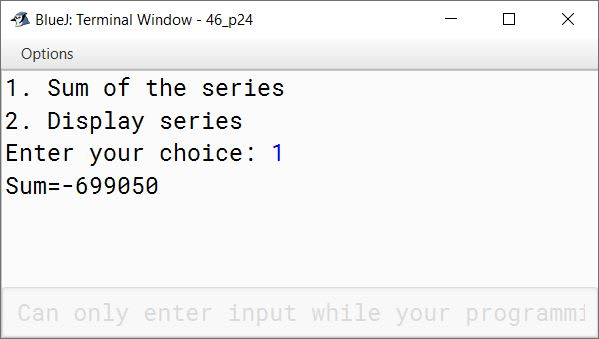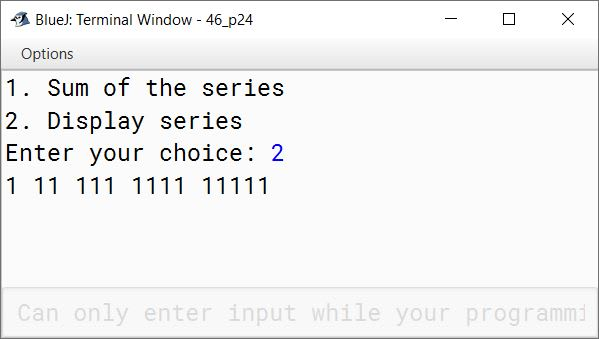# Iterative Constructs In Java

## Multiple Choice Questions

#### Question 1

When the statements are repeated sequentially a number of times in a program, the construct is known as:

1. iteration ✓
2. sequence
3. selection
4. none

#### Question 2

Which of the following statement is exit controlled loop?

1. for
2. while
3. do-while ✓
4. if-else

#### Question 3

Which of the following loop does not execute even once if condition is false in the beginning?

1. do-while
2. while ✓
3. for ✓
4. nested loop

#### Question 4

To execute a loop 10 times, which of the following statement satisfies:

1. for(i=6;i<=26;i=i+2)
2. for(i=3;i<=30;i=i+3) ✓
3. for(i=0;i<10;i=i++)
4. all of the above

#### Question 5

Which of the following statement uses multiple branches?

1. loop
2. continue
3. switch ✓
4. break

#### Question 6

Which of the following loop checks the condition first and then execution begins?

1. do-while
2. do
3. while loop ✓
4. for ✓

#### Question 7

To find the sum of whole numbers upto 10, a loop runs:

1. once
2. ten times
3. eleven times ✓
4. any number of times

#### Question 8

How many times the loop, for (i=1; ;i++), will execute, if there is no statement to terminate the loop?

1. 1
2. 0
3. infinite ✓
4. none

#### Question 9

Which of the following statements uses a case called default?

1. do-while
2. while
3. switch ✓
4. all of the above

#### Question 10

A loop statement is given as:

```for(i=10;i<10,i++)
{
Statement
}```

For how many times will the given loop statement be executed:

1. never ✓
2. 1 time
3. 10 times
4. infinite

#### Question 1

What do you understand by iterative process? How can it be resolved by using loop?
Iterative process means repeating a set of actions a certain number of times to perform some task. Loops in programming languages like Java enable us to repeat a single statement or a set of statements as long as the desired condition remains true.

#### Question 2

Explain for loop with an example.
for loop is an entry-controlled loop. Below is an example of for loop:

```for (int i = 1; i <= 12; i++) {
int a = 2 * i;
System.out.println("2 x " + i + "\t= " + a);
}```

This for loop prints the table of 2 till 12. `int i = 1` is the initialization part of the for loop, it is executed only once when the loop gets executed for the first time. `i <= 12` is the condition part of the for loop, it is executed before the start of each iteration. Loop iterates as long as this condition remains true. Once it becomes false, execution of the loop is stopped. `i++` is the update part of the for loop. It is executed at the end of each iteration.

#### Question 3

Name the different types of loop statements.

1. for
2. while
3. do-while

#### Question 4

What are the parameters needed to create a for loop?
The following parameters are commonly used in a for loop:

1. An initial value for the loop control variable.
2. A condition—loop will iterate as long as this condition remains true.
3. An update expression to modify the loop control variable after every iteration.
4. Body of the loop which consists of the statements that needs to be repeatedly executed.

#### Question 5

Define the following with their constructs:
(a) Entry controlled loop
An entry-controlled loop checks the condition at the time of entry. Only if the condition is true, the program control enters the body of the loop. for and while loops are entry-controlled loops.
(b) Exit controlled loop
An exit-controlled loop checks the condition after executing its body. If the condition is true, loop will perform the next iteration otherwise program control will move out of the loop. do-while loop is an exit-controlled loop.

#### Question 6

Write down the general format of:
(a) for loop

``````for (initialization; condition; update) {
//loop-body
}
``````

(b) do - while

``````do {
//loop-body
} while (condition);
``````

(c) while loop

``````while (condition) {
//loop-body
}
``````

#### Question 7

What is the purpose of using:
(a) break statement
break statement is used to unconditionally jump out of the loop
(b) continue statement
continue statement is used to unconditionally jump to the next iteration of the loop, skipping the remaining statements of the current iteration.

#### Question 8

What do you understand by inter-conversion of loops?
We can convert the repetitive logic written using one type of loop into any of the other 2 types. For example, if some repetitive logic is coded using a for loop, we can convert that for loop into while or do-while loop. This is termed inter-conversion of loops.

#### Question 9

What are the different ways to inter-convert the loops? Name them.

1. for loop to while loop
2. for loop to do-while loop
3. do-while loop to while loop
4. do-while loop to for loop
5. while loop to do-while loop
6. while loop to for loop

#### Question 10

Define the following:
(a) Finite loop
A loop which iterates for a finite number of iterations is termed as a finite loop.

(b) Delay loop
A loop which is used to pause the execution of the program for some finite amount of time is termed as Delay loop. Delay loops have an empty loop body.

(c) Infinite loop
A loop which continues iterating indefinitely and never stops is termed as infinite loop.

(d) Null loop
A loop which has an empty loop body is termed as a null loop.

#### Question 11

Distinguish between:

(a) for and while loop

1. for loop is a suitable choice when we know the number of iterations beforehand. while loop is helpful in situations where numbers of iterations is not known.
2. Omitting the condition in for loop will lead to an infinite loop whereas if condition is not provided in while loop, it will cause a compilation error.

(b) while and do-while loop

1. while is an entry-controlled loop whereas do-while is an exit-controlled loop
2. while loop is helpful in situations where numbers of iterations is not known. do-while is suitable when we need to display a menu to the user.

#### Question 12

State one difference and one similarity between while and do-while loop
Similarity — Both while and do-while are suitable in situations where numbers of iterations is not known.
Difference — while is an entry-controlled loop whereas do-while is an exit-controlled loop

#### Question 13

State one similarity and one difference between while and for loop.
Similarity — Both for and while are entry-controlled loops
Difference — for loop is a suitable choice when we know the number of iterations beforehand. while loop is helpful in situations where numbers of iterations is not known.

#### Question 14

Give two differences between Step loop and Continuous loop.
In Continuous loop, loop control variable is incremented or decremented by 1 in each iteration whereas in Step loop the loop control variable is incremented or decremented by more than 1 in each iteration.

## Predict the Output of the following Programs

#### Question 1

```class dkl
{
public static void main(String args[])
{
int i;
for(i = -1;i<10;i++)
{
System.out.println(++i);
}
}
}```
```0
2
4
6
8
10
```
##### Explanation

This table shows the changes in the value of i as the for loop iterates:

iRemarks
-1Initial value
01st Iteration — i is -1, ++i makes it 0
22nd Iteration — i becomes 1, ++i makes it 2
43rd Iteration — i becomes 3, ++i makes it 4
64th Iteration — i becomes 5, ++i makes it 6
85th Iteration — i becomes 7, ++i makes it 8
106th Iteration — i becomes 9, ++i makes it 10
11Once i becomes 11, condition is false and loop stops iterating.

#### Question 2

```class dk2
{
public static void main(String args[])
{
int i=2,k=1;
while (++i<6)
k *= i;
System.out.println(k);
}
}```
```60
```
##### Explanation

This table shows the change in values of i and k as while loop iterates:

ikRemarks
21Initial values
331st Iteration
4122nd Iteration
5603rd Iteration
660Once i becomes 6, condition is false and loop stops iterating.

Notice that `System.out.println(k);` is not inside while loop. As there are no curly braces so only the statement `k *= i;` is inside the loop. The statement `System.out.println(k);` is outside the while loop, it is executed once and prints value of k which is 60 to the console.

#### Question 3

```class dk3
{
public static void main(String args[])
{
int m=2,n=15;
for(int i=1;i<=5;i++)
{
m++;--n;
System.out.println("m="+m);
System.out.println("n="+n);
}
}
}```
```m=3
n=14
m=4
n=13
m=5
n=12
m=6
n=11
m=7
n=10
```
##### Explanation

This table shows the change in values of m, n and i as the for loop iterates:

mniRemarks
215Initial values
31411st Iteration
41322nd Iteration
51233rd Iteration
61144th Iteration
71055th Iteration
7106Once i becomes 6, condition is false and loop stops iterating.

#### Question 4

Determine how many times the body of the loop will be executed.

```class dk4
{
public static void main(String args[])
{
int x=5,y=50;
while(x<=y)
{
y=y/x;
System.out.println(y);
}
}
}```
##### Output
```10
2
```

The loop will execute 2 times

##### Explanation
xyRemarks
550Initial values
510After 1st iteration
52After 2nd iteration

After 2 iterations y becomes less than x so condition of while loop becomes false and it stops executing.

## Rewrite the following Programs

#### Question 1

Using do while:

```class Test
{
public static void main(String args[])
{
int x,c;
for(x=10,c=20;c>=10;c=c-2)
{
x++;
System.out.println(x);
}
}
}```
##### Solution
```class Test
{
public static void main(String args[])
{
int x=10, c=20;
do {
x++;
System.out.println(x);
c=c-2;
} while (c>=10);
}
}```

#### Question 2

Using do while:

```class Pattern
{
public static void main(String args[])
{
int i,j;
for(i=5;i>=1;i--)
{
System.out.print(i);
}
System.out.println();
}
}```
##### Solution
```class Pattern
{
public static void main(String args[])
{
int i=5,j;
do {
System.out.print(i);
i--;
} while (i>=1);
System.out.println();
}
}```

#### Question 3

Using do while:

```class Number
{
public static void main(String args[])
{
int i,n=191,c=0;
for(i=1;i<=n;i++)
{
if(n%i==0)
c=c+1;
}
if(c==2)
System.out.println("Prime");
else
System.out.println("Not Prime");
}
}```
##### Solution
```class Number
{
public static void main(String args[])
{
int i=1,n=191,c=0;
do {
if(n%i==0)
c=c+1;
i++;
} while (i<=n);
if(c==2)
System.out.println("Prime");
else
System.out.println("Not Prime");
}
}```

#### Question 4

Using while loop:

```import java.io.*;
class Sample
{
public static void main(String args[]) throws IOException
{
int n,r;
System.out.println("Enter a number");
do
{
r=n%10;
n=n/10;
System.out.println(r);
}
while(n!=0);
}
}```
##### Solution
```import java.io.*;
class Sample
{
public static void main(String args[]) throws IOException
{
int n,r;
System.out.println("Enter a number");
while(n!=0)
{
r=n%10;
n=n/10;
System.out.println(r);
}
}
}```

## Solutions to Unsolved Java Programs

#### Question 1

Write the programs in Java to display the first ten terms of the following series:

(a) 1, 4, 9, 16,

```public class KboatSeries
{
public static void main(String args[]) {
for (int i = 1; i <= 10; i++) {
System.out.print(i * i + " ");
}
}
}```
##### Output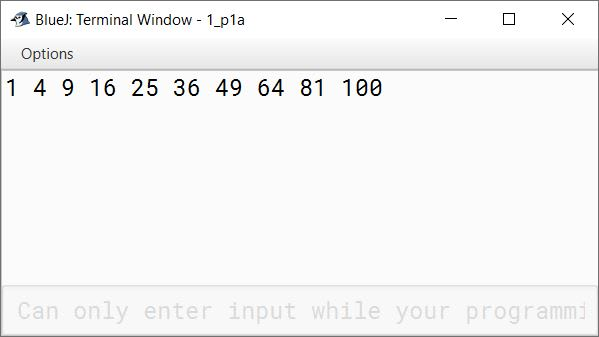(b) 1, 2, 4, 7, 11,

```public class KboatSeries
{
public static void main(String args[]) {
for (int i = 0; i < 10; i++) {
int term = 1 + ((i * (i + 1)) / 2);
System.out.print(term + " ");
}
}
}```
##### Output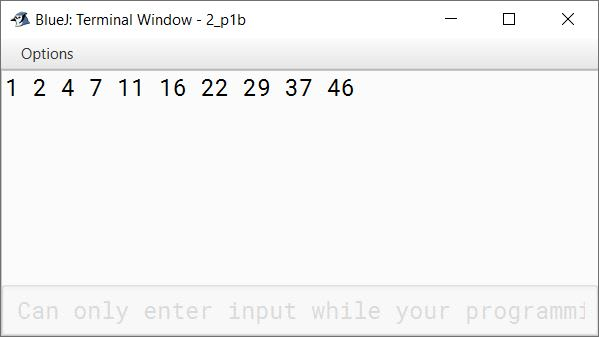(c) 3, 6, 9, 12,

```public class KboatSeries
{
public static void main(String args[]) {
for (int i = 3; i <= 30; i = i + 3) {
System.out.print(i + " ");
}
}
}```
##### Output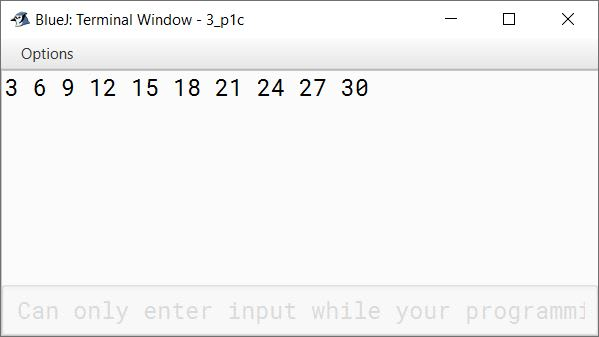(d) 4, 8, 16, 32,

```public class KboatSeries
{
public static void main(String args[]) {
for (int i = 2; i <= 11; i++) {
System.out.print((int)(Math.pow(2, i)) + " ");
}
}
}```
##### Output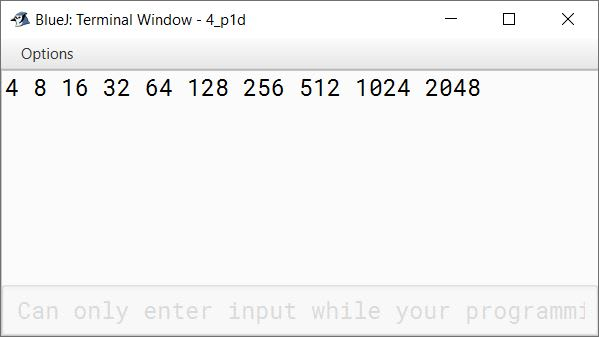(e) 1.5, 3.0, 4.5, 6.0,

```public class KboatSeries
{
public static void main(String args[]) {
float term = 1.5f;
for (int i = 1; i <= 10; i++) {
System.out.print(term + " ");
term += 1.5f;
}
}
}```
##### Output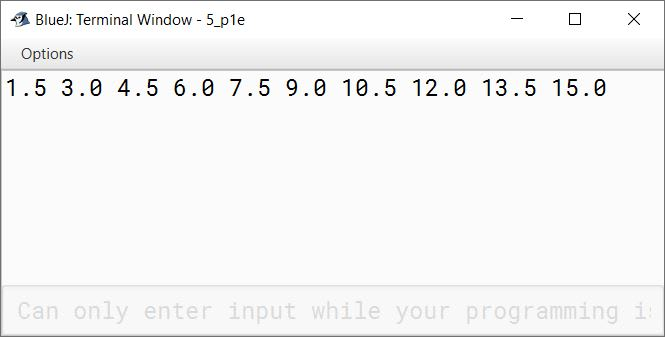(f) 0, 7, 26,

```public class KboatSeries
{
public static void main(String args[]) {
for (int i = 1; i <= 10; i++) {
int term = (int)(Math.pow(i, 3) - 1);
System.out.print(term + " ");
}
}
}```
##### Output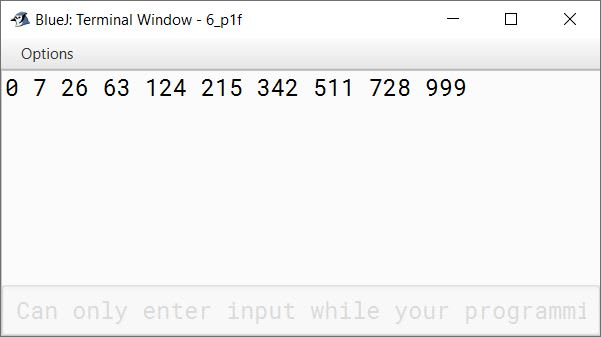(g) 1, 9, 25, 49,

```public class KboatSeries
{
public static void main(String args[]) {
for (int i = 1; i <= 19; i = i + 2) {
System.out.print((i * i) + " ");
}
}
}```
##### Output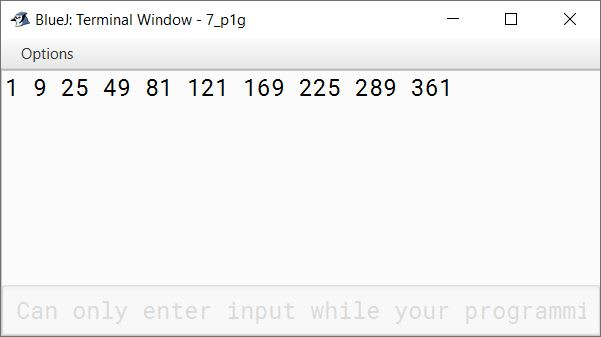(h) 4, 16, 36, 64,

```public class KboatSeries
{
public static void main(String args[]) {
for (int i = 2; i <= 20; i = i + 2) {
System.out.print((i * i) + " ");
}
}
}```
##### Output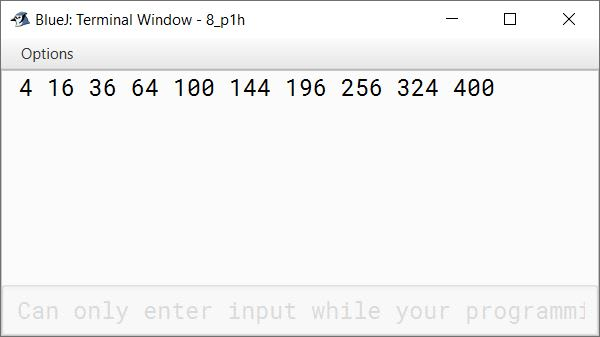(i) 0, 3, 8, 15,

```public class KboatSeries
{
public static void main(String args[]) {
for (int i = 1; i <= 10; i++) {
System.out.print((i * i - 1) + " ");
}
}
}```
##### Output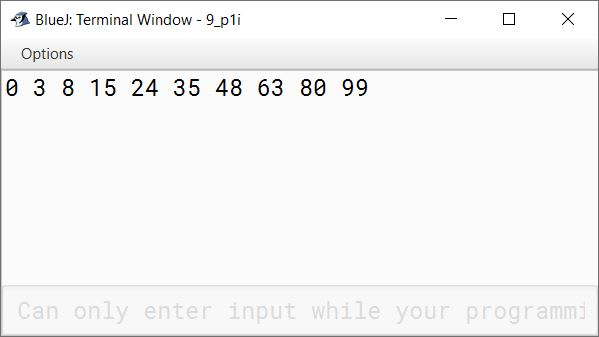(j) 24, 99, 224, 399,

```public class KboatSeries
{
public static void main(String args[]) {
for (int i = 5; i <= 50; i = i + 5) {
int term = (int)(Math.pow(i, 2) - 1);
System.out.print(term + " ");
}
}
}```
##### Output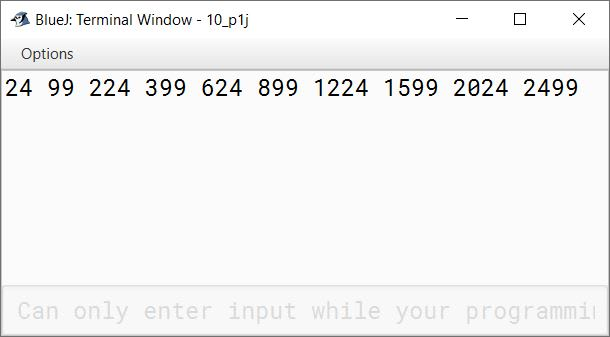(k) 2, 5, 10, 17,

```public class KboatSeries
{
public static void main(String args[]) {
for (int i = 1; i <= 10; i++) {
System.out.print((i * i + 1) + " ");
}
}
}```
##### Output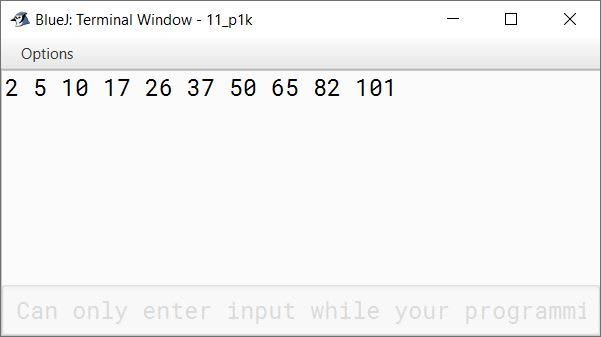#### Question 2

Write a program to input any 50 numbers (including positive and negative).
(a) Count the positive numbers
(b) Count the negative numbers
(c) Sum of positive numbers
(d) Sum of negative numbers

```import java.util.Scanner;

public class KboatIntegers
{
public static void main(String args[]) {
Scanner in = new Scanner(System.in);
int pSum = 0, pCount = 0, nSum = 0, nCount = 0;
System.out.println("Enter 50 numbers");

for (int i = 1; i <= 50; i++) {
int n = in.nextInt();
if (n >= 0) {
pSum += n;
pCount++;
}
else {
nSum += n;
nCount++;
}
}

System.out.println("Positive Count = " + pCount);
System.out.println("Positive Sum = " + pSum);
System.out.println("Negative Count = " + nCount);
System.out.println("Negative Sum = " + nSum);
}
}```
##### Output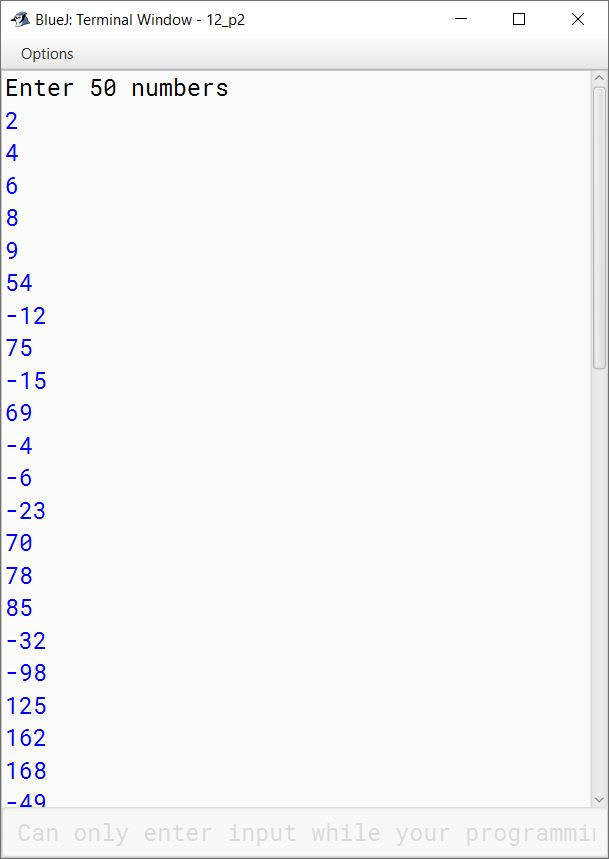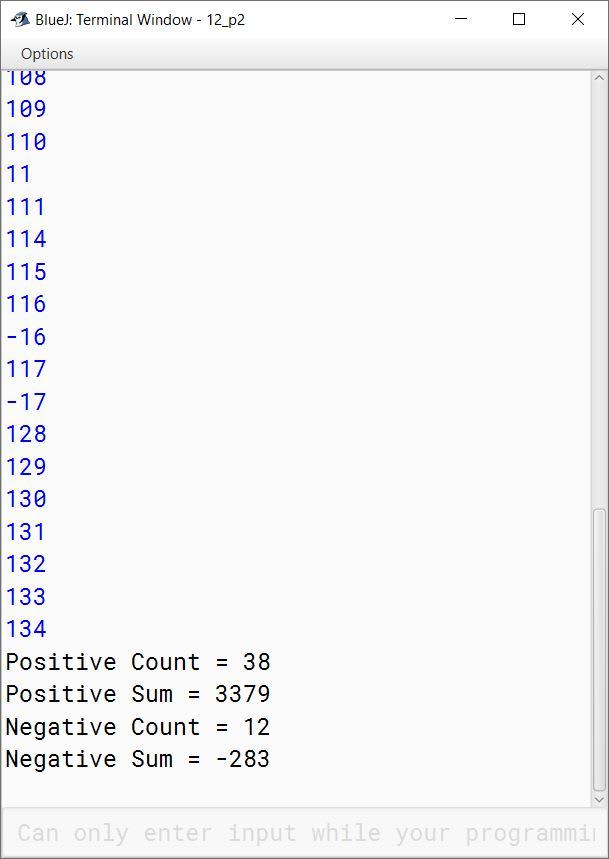#### Question 3

Write a program to calculate the sum of all odd numbers and even numbers between a range of numbers from m to n (both inclusive) where m < n. Input m and n (where m<n).

```import java.util.Scanner;

public class KboatOddEvenSum
{
public static void main(String args[]) {
Scanner in = new Scanner(System.in);
System.out.print("Enter m: ");
int m = in.nextInt();
System.out.print("Enter n: ");
int n = in.nextInt();
long sumOdd = 0, sumEven = 0;

if (m > n) {
System.out.println("m should be less than n");
}
else {
for (int i = m; i <=n; i++) {
if (i % 2 == 0)
sumEven += i;
else
sumOdd += i;
}

System.out.println("Even Sum: " + sumEven);
System.out.println("Odd Sum: " + sumOdd);
}
}
}```
##### Output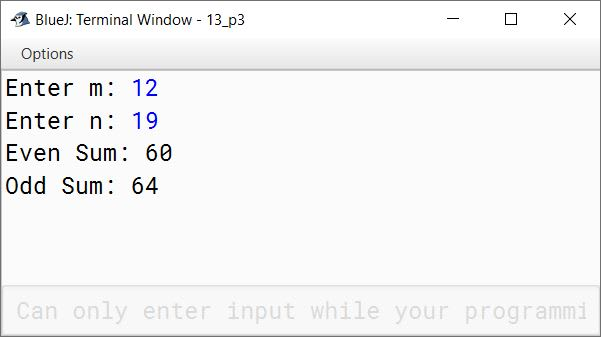#### Question 4

Write a program to enter any 50 numbers and check whether they are divisible by 5 or not. If divisible then perform the following tasks:
(a) Display all the numbers ending with the digit 5.
(b) Count those numbers ending with 0 (zero).

```import java.util.Scanner;

public class KboatDivisibleBy5
{
public static void main(String args[]) {
Scanner in = new Scanner(System.in);
int n, c = 0;
System.out.println("Enter 50 numbers");
for (int i = 1; i <= 50; i++) {
n = in.nextInt();
if (n % 5 == 0) {
if (n % 10 == 5)
System.out.println("Number end with the digit 5");
if (n % 10 == 0)
c++;
}
}
System.out.println("Count of numbers ending with 0: " + c);
}
}```
##### Output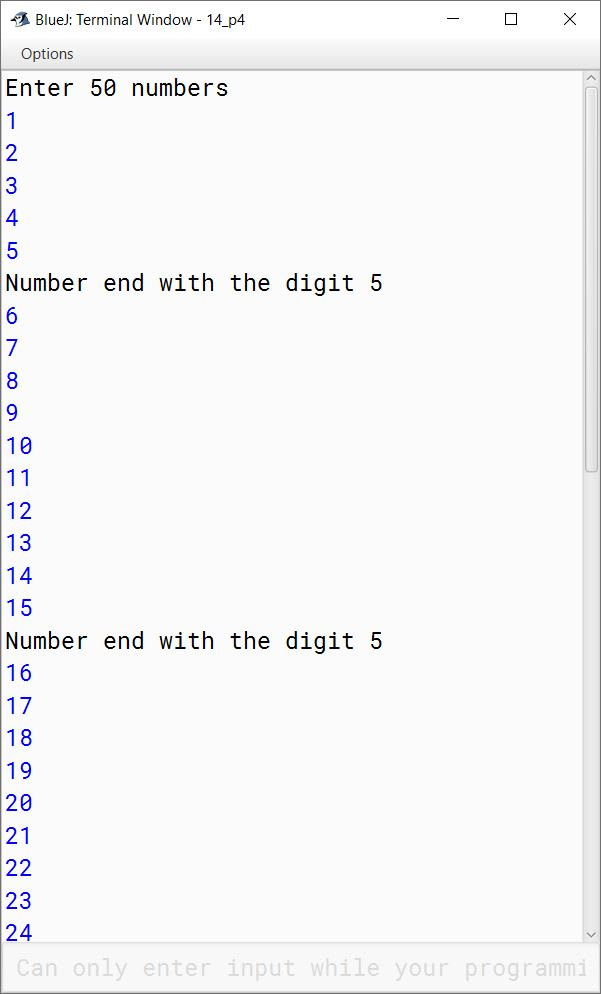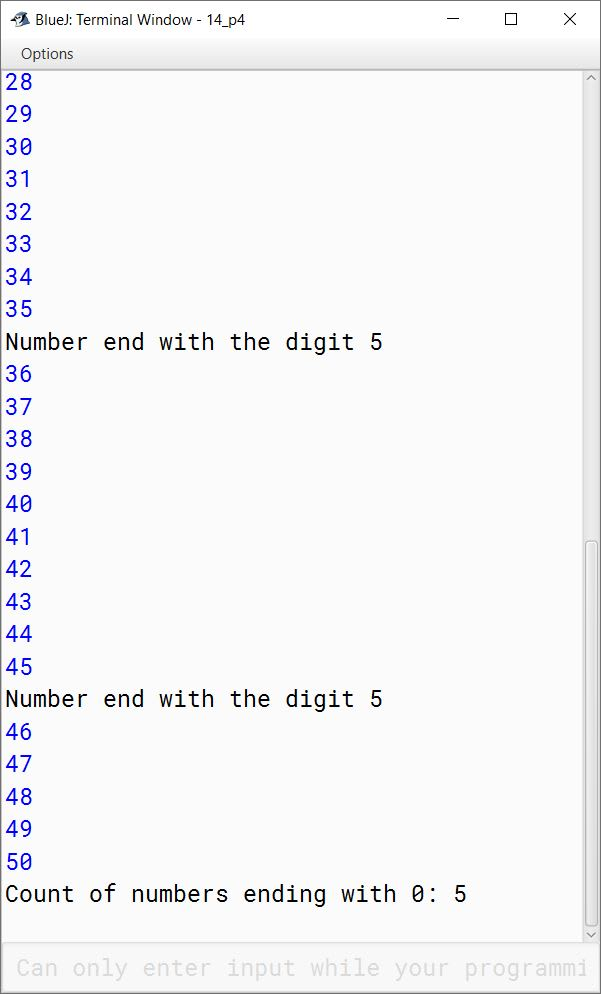#### Question 5

Write a program to display all the numbers between m and n input from the keyboard (where m<n, m>0, n>0), check and print the numbers that are perfect square. e.g. 25, 36, 49, are said to be perfect square numbers.

```import java.util.Scanner;

public class KboatPerfectSquare
{
public static void main(String args[]) {
Scanner in = new Scanner(System.in);
System.out.print("Enter m: ");
int m = in.nextInt();
System.out.print("Enter n: ");
int n = in.nextInt();

if (m < n && m > 0 && n > 0) {
for (int i = m; i <= n; i++) {
System.out.println("Number = " + i);
double sroot = Math.sqrt(i);
if (sroot == Math.floor(sroot))
System.out.println(i + " is a perfect square");
}
}
else {
System.out.println("Invalid input");
}
}
}```
##### Output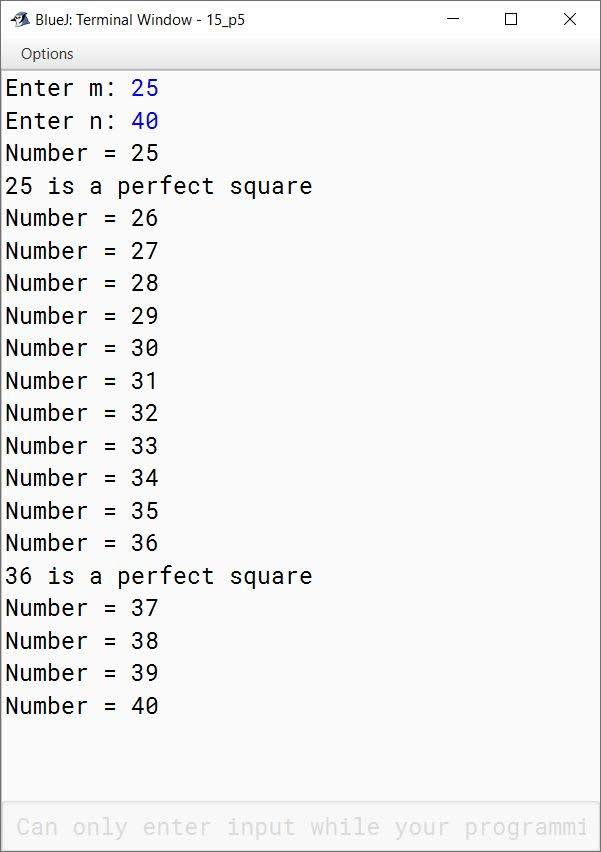#### Question 6

Write a program to display all the 'Buzz Numbers' between p and q (where p<q). A 'Buzz Number' is the number which ends with 7 or is divisible by 7.

```import java.util.Scanner;

public class KboatBuzzNumber
{
public static void main(String args[]) {
Scanner in = new Scanner(System.in);
System.out.print("Enter p: ");
int p = in.nextInt();
System.out.print("Enter q: ");
int q = in.nextInt();
if (p < q) {
System.out.println("Buzz Numbers between "
+ p + " and " + q);
for (int i = p; i <= q; i++) {
if (i % 10 == 7 || i % 7 == 0)
System.out.println(i);
}
}
else {
System.out.println("Invalid Inputs!!!");
System.out.println("p should be less than q");
}

}
}```
##### Output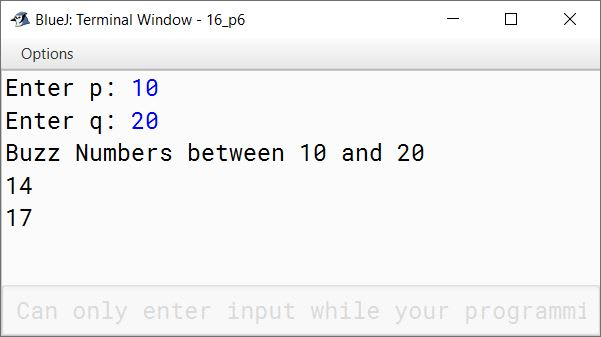#### Question 7

Write a program to input marks in English, Maths and Science of 40 students who have passed ICSE Examination 2014. Now, perform the following tasks:
(a) Number of students, who have secured 95% or more in all the subjects.
(b) Number of students, who have secured 90% or more in English, Maths and Science.

```import java.util.Scanner;

public class KboatExamResult
{
public static void main(String args[]) {
Scanner in = new Scanner(System.in);
int ta = 0, te = 0, tm = 0, ts = 0;
for (int i = 1; i <= 40; i++) {
System.out.println("Enter marks of student " + i);
System.out.print("English: ");
int eng = in.nextInt();
System.out.print("Maths: ");
int maths = in.nextInt();
System.out.print("Science: ");
int sci = in.nextInt();

if (eng >= 95 && maths >= 95 && sci >= 95)
ta++;

if (eng >= 90)
te++;

if (maths >= 90)
tm++;

if (sci >= 90)
ts++;
}
System.out.println("No. of students >= 95% in all subjects: " + ta);
System.out.println("No. of students >= 90% in English: " + te);
System.out.println("No. of students >= 90% in Maths: " + tm);
System.out.println("No. of students >= 90% in Science: " + ts);
}
}```
##### Output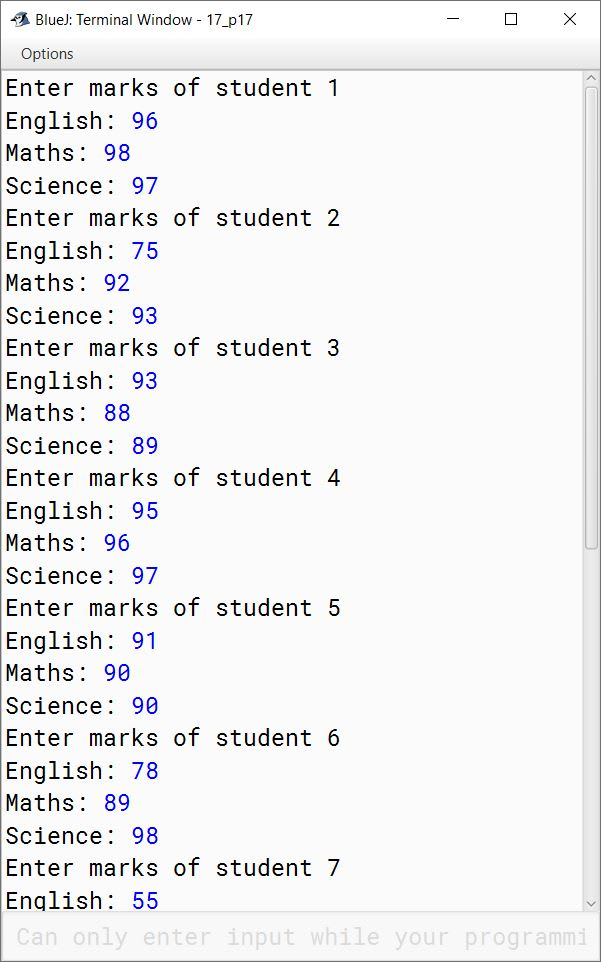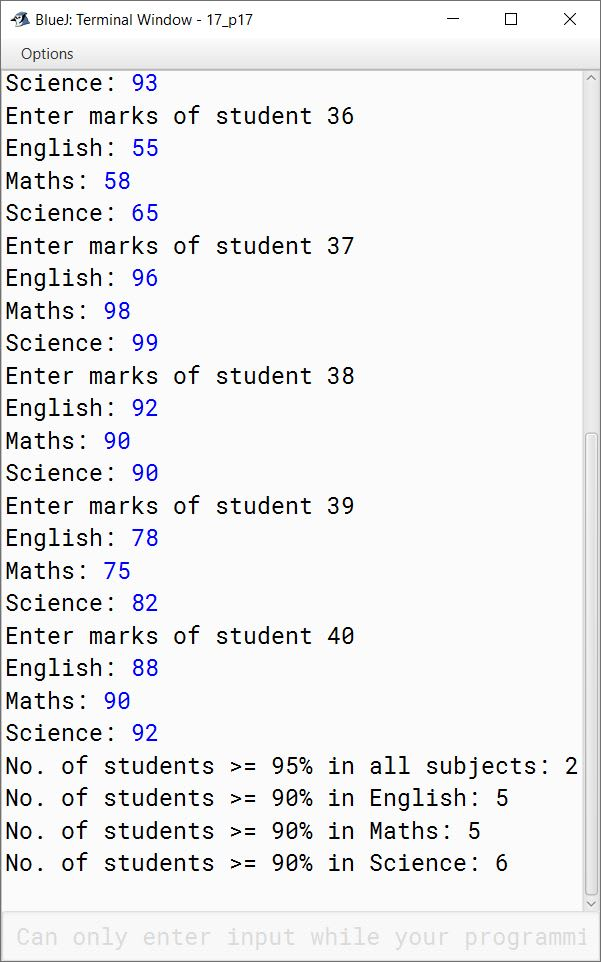#### Question 8

Write a program in Java to find the sum of the given series :
(a) 1 + 4 + 9 + ...... + 400

```public class KboatSeries
{
public static void main(String args[]) {
int sum = 0;
for (int i = 1; i <= 20; i++)
sum += (i*i);
System.out.println("Sum = " + sum);
}
}```
##### Output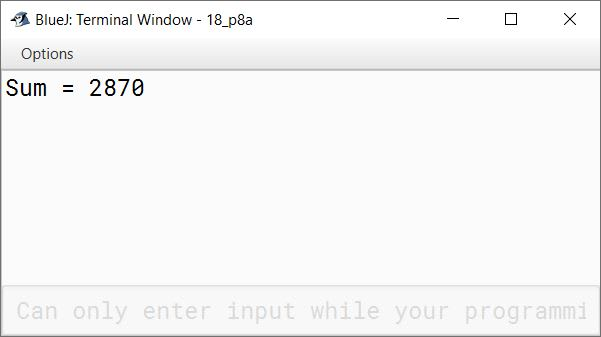(b) 1 + (1/2) + (1/3) + ...... + (1/20)

```public class KboatSeries
{
public static void main(String args[]) {
double sum = 0.0;
for (int i = 1; i <= 20; i++)
sum += (1.0 / i);
System.out.println("Sum = " + sum);
}
}```
##### Output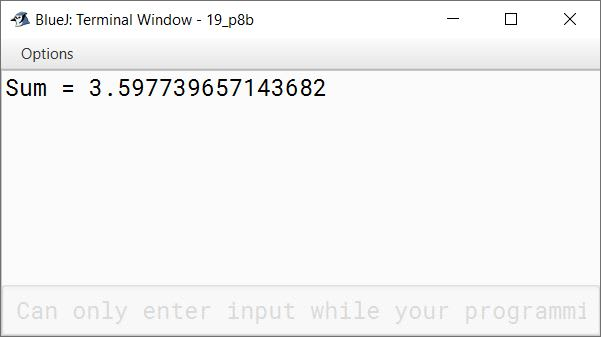(c) 1 + (1/3) + (1/5) + ...... + (1/19)

```public class KboatSeries
{
public static void main(String args[]) {
double sum = 0.0;
for (int i = 1; i <= 19; i = i + 2)
sum += (1.0 / i);
System.out.println("Sum = " + sum);
}
}```
##### Output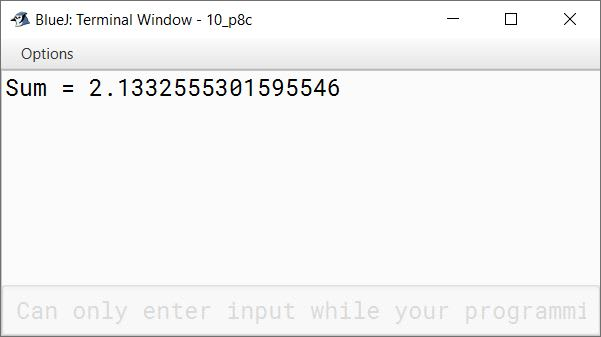(d) (1/2) + (2/3) + (3/4) + ...... + (19/20)

```public class KboatSeries
{
public static void main(String args[]) {
double sum = 0.0;
for (int i = 1; i <= 19; i++)
sum += (i / (double)(i + 1));
System.out.println("Sum = " + sum);
}
}```
##### Output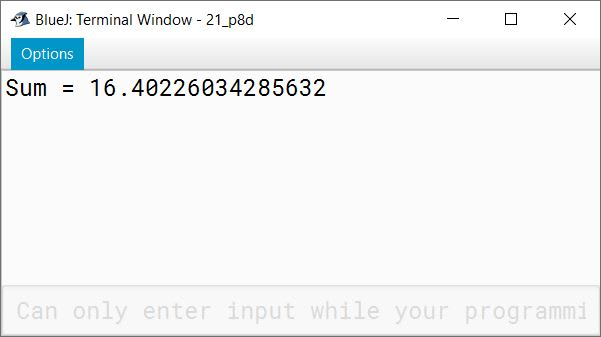(e) 2 - 4 + 6 - 8 + ...... - 20

```public class KboatSeries
{
public static void main(String args[]) {
double sum = 0.0;
for (int i = 1; i <= 10; i++) {
if (i % 2 == 0)
sum -= i * 2;
else
sum += i * 2;
}
System.out.println("Sum = " + sum);
}
}```
##### Output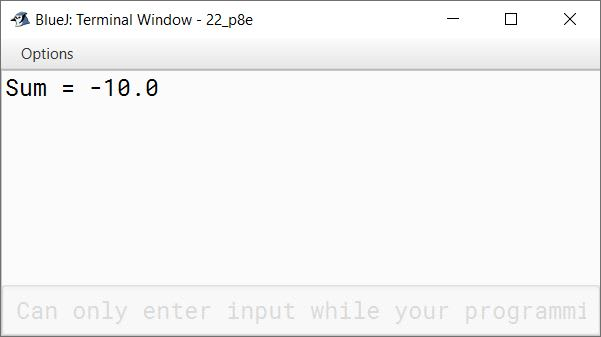(f) (1*2) + (2*3) + ...... + (19*20)

```public class KboatSeries
{
public static void main(String args[]) {
int sum = 0;
for (int i = 1; i <= 19; i++)
sum += i * (i + 1);
System.out.println("Sum = " + sum);
}
}```
##### Output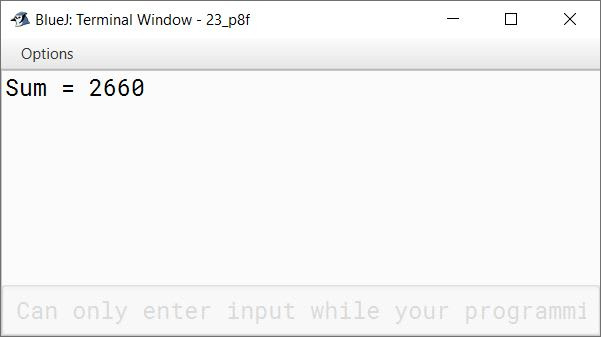#### Question 9

Write a program to input a number and count the number of digits. The program further checks whether the number contains odd number of digits or even number of digits.
Sample Input: 749
Sample Output: Number of digits=3
The number contains odd number of digits.

```import java.util.Scanner;

public class KboatDigitCount
{
public static void main(String args[]) {
Scanner in = new Scanner(System.in);
System.out.print("Enter number: ");
int n = in.nextInt();
int dc = 0;

while (n != 0) {
dc++;
n /= 10;
}

System.out.println("Number of digits = " + dc);

if (dc % 2 == 0)
System.out.println("The number contains even number of digits");
else
System.out.println("The number contains odd number of digits");
}
}```
##### Output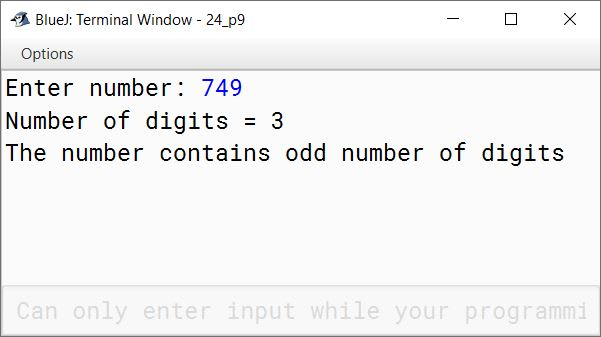#### Question 10

Write a program to input a number and display the new number after reversing the digits of the original number. The program also displays the absolute difference between the original number and the reversed number.
Sample Input: 194
Sample Output: 491
Absolute Difference= 297

```import java.util.Scanner;

public class KboatDigitReverse
{
public static void main(String args[]) {

Scanner in = new Scanner(System.in);
System.out.print("Enter Number: ");
int orgNum = in.nextInt();

int copyNum = orgNum;
int revNum = 0;

while(copyNum != 0) {
int digit = copyNum % 10;
copyNum /= 10;
revNum = revNum * 10 + digit;
}

int diff = revNum - orgNum;
System.out.println("Reversed Number = " + revNum);
System.out.println("Absolute Difference = " + Math.abs(diff));
}
}```
##### Output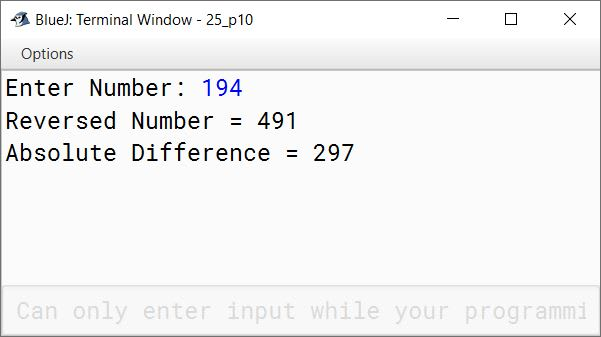#### Question 11

The Greatest Common Divisor (GCD) of two integers is calculated by the continued division method. Divide the larger number by the smaller, the remainder then divides the previous divisor. The process repeats unless the remainder reaches to zero. The last divisor results in GCD.
Sample Input: 45, 20
Sample Output: GCD=5

```import java.util.Scanner;

public class KboatGCD
{
public static void main(String args[]) {
Scanner in = new Scanner(System.in);
System.out.print("Enter first number: ");
int a = in.nextInt();
System.out.print("Enter second number: ");
int b = in.nextInt();
while (b != 0) {
int t = b;
b = a % b;
a = t;
}
System.out.println("GCD=" + a);
}
}```
##### Output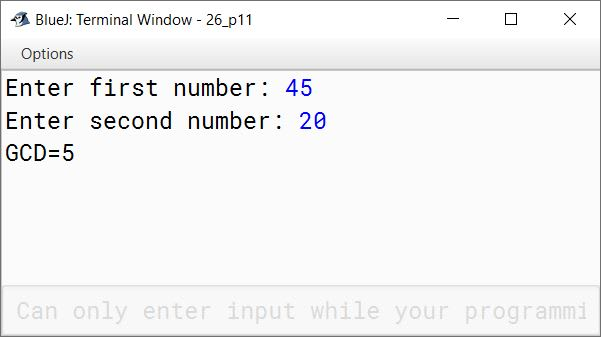#### Question 12

Write a program in Java to find the sum of the given series :

(a) S = a2 + a2 / 2 + a2 / 3 + ...... + a2 / 10

```import java.util.Scanner;

public class KboatSeries
{
public static void main(String args[]) {
Scanner in = new Scanner(System.in);
System.out.print("Enter a: ");
int a = in.nextInt();
double sum = 0.0;
for (int i = 1; i <= 10; i++)
sum += Math.pow(a, 2) / i;
System.out.println("Sum = " + sum);
}
}```
##### Output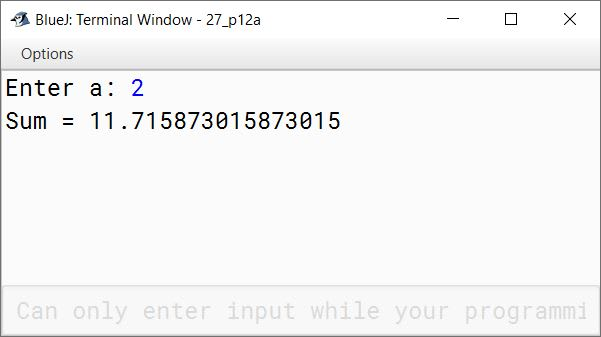(b) S = a + a2 / 2 + a3 / 3 + ...... + a10 / 10

```import java.util.Scanner;

public class KboatSeries
{
public static void main(String args[]) {
Scanner in = new Scanner(System.in);
System.out.print("Enter a: ");
int a = in.nextInt();
double sum = 0.0;
for (int i = 1; i <= 10; i++)
sum += Math.pow(a, i) / i;
System.out.println("Sum = " + sum);
}
}```
##### Output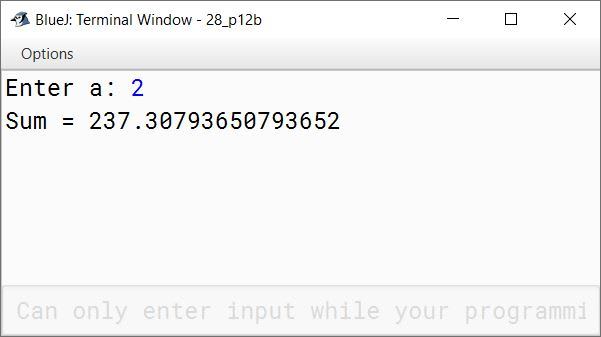(c) S = (a*2) + (a*3) + ...... + (a*20)

```import java.util.Scanner;

public class KboatSeries
{
public static void main(String args[]) {
Scanner in = new Scanner(System.in);
System.out.print("Enter a: ");
int a = in.nextInt();
long sum = 0;
for (int i = 2; i <= 20; i++)
sum += a * i;
System.out.println("Sum = " + sum);
}
}```
##### Output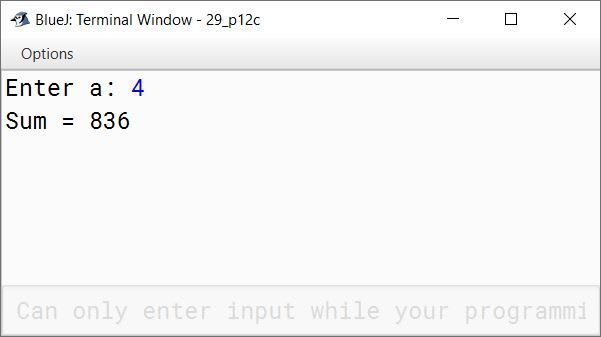(d) S = a + a2 + a3 + ...... + an

```import java.util.Scanner;

public class KboatSeries
{
public static void main(String args[]) {
Scanner in = new Scanner(System.in);
System.out.print("Enter a: ");
int a = in.nextInt();
System.out.print("Enter n: ");
int n = in.nextInt();
long sum = 0;
for (int i = 1; i <= n; i++)
sum += Math.pow(a, i);
System.out.println("Sum = " + sum);
}
}```
##### Output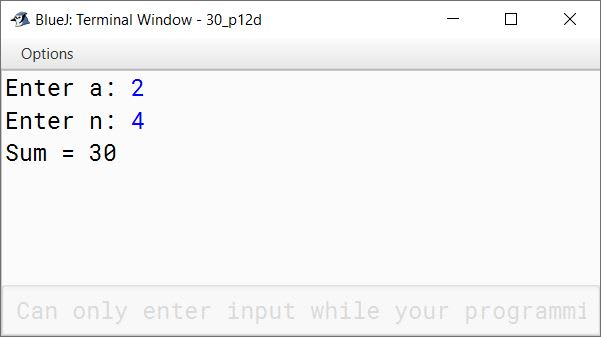(e) S = 1 + 22 / a + 33 / a2 + ...... to n terms

```import java.util.Scanner;

public class KboatSeries
{
public static void main(String args[]) {
Scanner in = new Scanner(System.in);
System.out.print("Enter a: ");
int a = in.nextInt();
System.out.print("Enter n: ");
int n = in.nextInt();
double sum = 1.0;
for (int i = 2; i <= n; i++)
sum += Math.pow(i, i) / Math.pow(a, i - 1);
System.out.println("Sum = " + sum);
}
}```
##### Output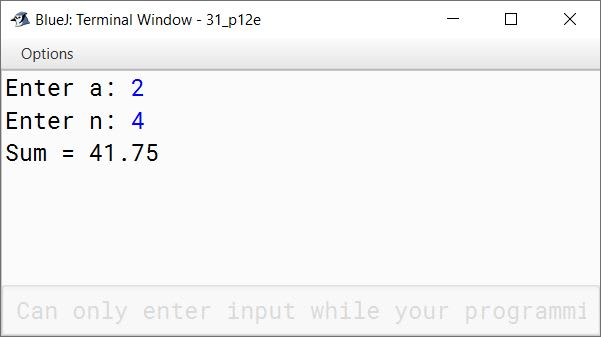(f) S = 12/a + 32 / a2 + 52 / a3 + ...... to n terms

```import java.util.Scanner;

public class KboatSeries
{
public static void main(String args[]) {
Scanner in = new Scanner(System.in);
System.out.print("Enter a: ");
int a = in.nextInt();
System.out.print("Enter n: ");
int n = in.nextInt();
double sum = 0.0;
for (int i = 1, j = 1; i <= n; i++, j=j+2)
sum += Math.pow(j, 2) / Math.pow(a, i);
System.out.println("Sum = " + sum);
}
}```
##### Output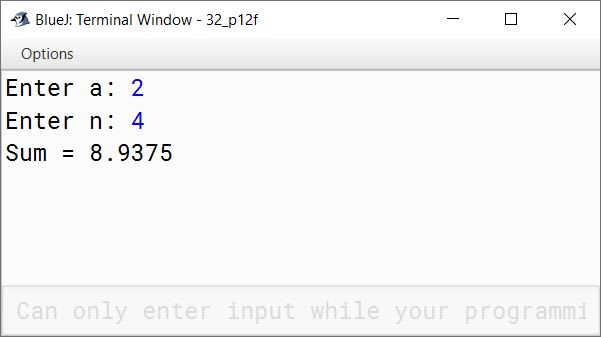(g) S = 1/a + 1/a2 + 1/a3 + ...... + 1/an

```import java.util.Scanner;

public class KboatSeries
{
public static void main(String args[]) {
Scanner in = new Scanner(System.in);
System.out.print("Enter a: ");
int a = in.nextInt();
System.out.print("Enter n: ");
int n = in.nextInt();
double sum = 0.0;
for (int i = 1; i <= n; i++)
sum += 1 / Math.pow(a, i);
System.out.println("Sum = " + sum);
}
}```
##### Output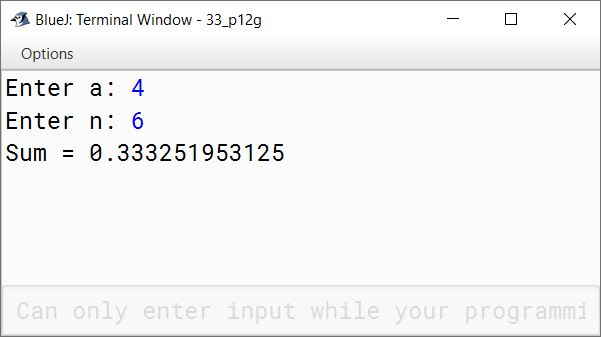(h) S = x/2 + x/5 + x/8 + x/11 + ...... + x/20

```import java.util.Scanner;

public class KboatSeries
{
public static void main(String args[]) {
Scanner in = new Scanner(System.in);
System.out.print("Enter x: ");
int x = in.nextInt();
double sum = 0.0;
for (int i = 2; i <= 20; i = i+3)
sum += (double)x / i;
System.out.println("Sum = " + sum);
}
}```
##### Output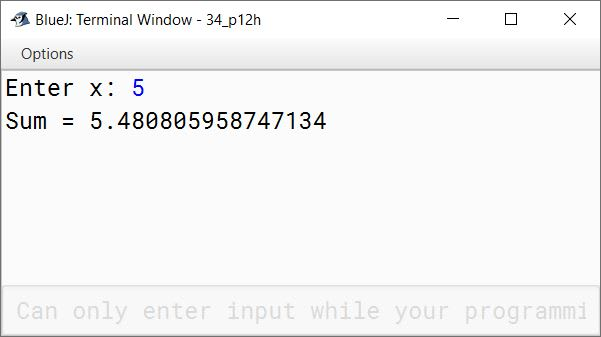#### Question 13

In order to reach the top of a pole, a monkey in his first attempt reaches to a height of 5 feet and in the subsequent jumps, he slips down by 2% of the height attained in the previous jump. The process repeats and finally the monkey reaches the top of the pole. Write a program to input height of the pole. Calculate and display the number of attempts the monkey makes to reach the top of the pole.

```import java.util.Scanner;

public class KboatMonkeyPole
{
public static void main(String args[]) {
Scanner in = new Scanner(System.in);
System.out.print("Enter height of the pole: ");
double poleHt = in.nextDouble();
double jumpHt = 5.0;
int numAttempts = 1;
while (jumpHt < poleHt) {
jumpHt += 5.0;
jumpHt -= 2 * jumpHt / 100.0;
numAttempts++;
}
System.out.println("Number of Attempts = " + numAttempts);
}
}```
##### Output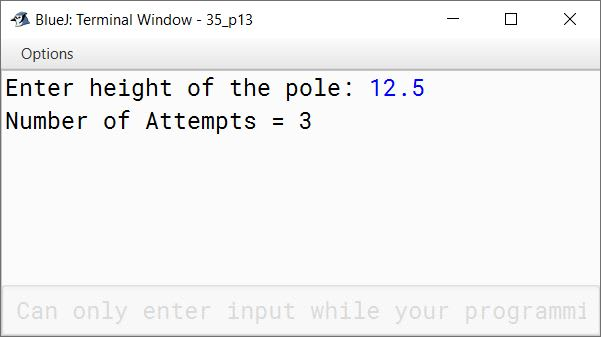#### Question 14

Write a program to input Principal (p), Rate (r) and Time (t). Calculate and display the amount, which is compounded annually for each year by using the formula:
Simple Interest (si) = (prt) / 100
p = p + si
[Hint: The amount after each year is the Principal for the next year]

```import java.util.Scanner;

public class KboatCompoundInterest
{
public static void main(String args[]) {
Scanner in = new Scanner(System.in);
System.out.print("Enter Principal: ");
double p = in.nextDouble();
System.out.print("Enter Rate: ");
double r = in.nextDouble();
System.out.print("Enter Time: ");
int t = in.nextInt();
double amt = p;
for (int i = 1; i <= t; i++) {
double interest = (amt * r * 1) / 100.0;
amt += interest;
System.out.println("Amount after " + i
+ " year = " + amt);
}
}
}```
##### Output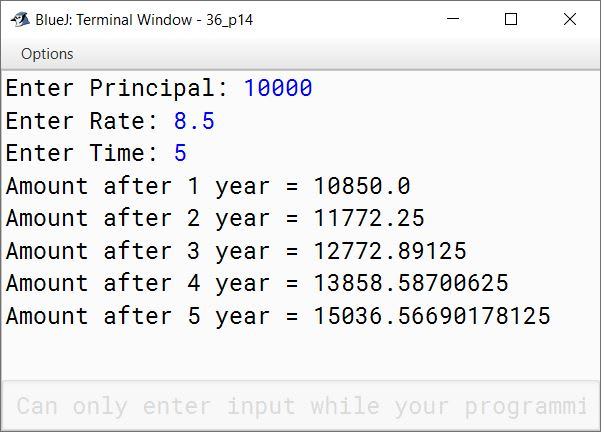#### Question 15

Write a menu driven program to input two positive numbers m and n (where m>n) and perform the following tasks:
(a) Find the sum of two numbers without using '+' operator.
(b) Find the product of two numbers without using '*' operator.
(c) Find the quotient and remainder of two numbers without using '/' and '%' operator.

[Hint: The last value obtained after each subtraction is the remainder and the number of iterations results in quotient.]
Sample Input: m=5, n=2
5 - 2 =3
3 - 2 = 1, thus Quotient = 2 and Remainder = 1

```import java.util.Scanner;

public class KboatNumberOperations
{
public static void main(String args[]) {
Scanner in = new Scanner(System.in);
System.out.println("1. Sum without '+' operator");
System.out.println("2. Product without '*' operator");
System.out.println("3. Quotient and Remainder without '/' & '%' operators");
int choice = in.nextInt();
System.out.print("Enter m: ");
int m = in.nextInt();
System.out.print("Enter n: ");
int n = in.nextInt();

if (m > n) {
switch (choice) {
case 1:
while (n > 0) {
m++;
n--;
}
System.out.println("Sum = " + m);
break;

case 2:
int p = 0;
while (n > 0) {
p += m;
n--;
}
System.out.println("Product = " + p);
break;

case 3:
int q = 0;
while (m >= n) {
m = m - n;
q++;
}
System.out.println("Quotient = " + q);
System.out.println("Remainder = " + m);
break;

default:
System.out.println("Incorrect Choice");
break;
}
}
else {
System.out.println("Invalid Inputs");
}
}
}```
##### Output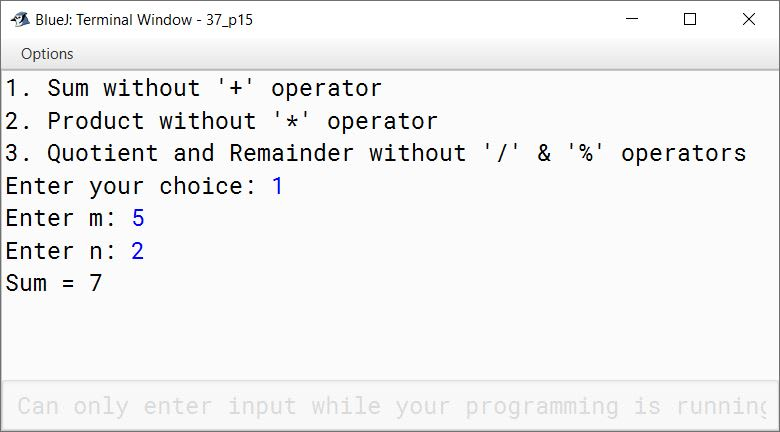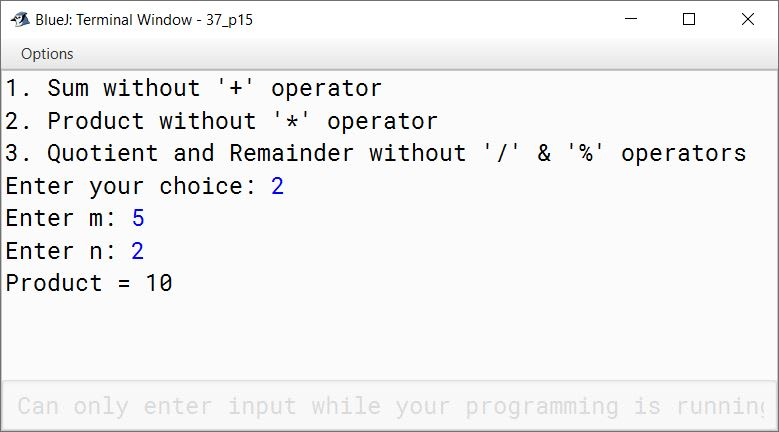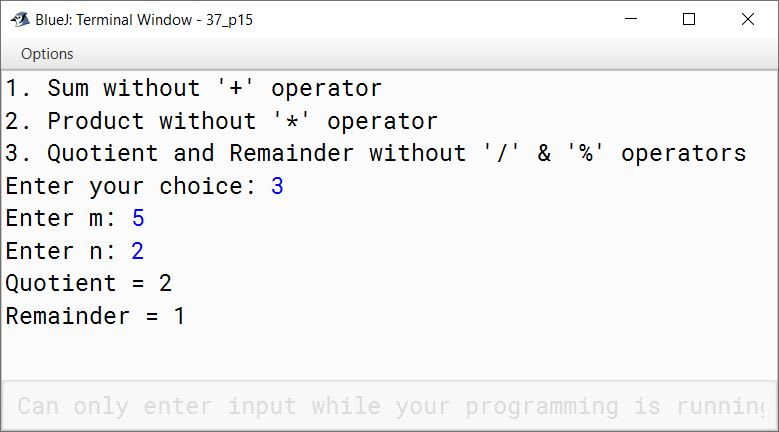#### Question 16

Write a menu driven class to accept a number from the user and check whether it is a Palindrome or a Perfect number.
(a) Palindrome number: (A number is a Palindrome which when read in reverse order is same as in the right order)
Example: 11, 101, 151 etc.
(b) Perfect number: (A number is called Perfect if it is equal to the sum of its factors other than the number itself.)
Example: 6 = 1 + 2 + 3

```import java.util.Scanner;

public class KboatPalinOrPerfect
{
public static void main(String args[]) {
Scanner in = new Scanner(System.in);
System.out.println("1. Palindrome number");
System.out.println("2. Perfect number");
int choice = in.nextInt();
System.out.print("Enter number: ");
int num = in.nextInt();

switch (choice) {
case 1:
int copyNum = num;
int revNum = 0;

while(copyNum != 0) {
int digit = copyNum % 10;
copyNum /= 10;
revNum = revNum * 10 + digit;
}

if (revNum == num)
System.out.println(num + " is palindrome");
else
System.out.println(num + " is not palindrome");
break;

case 2:
int sum = 0;

for (int i = 1; i <= num / 2; i++) {
if (num % i == 0) {
sum += i;
}
}

if (num == sum)
System.out.println(num + " is a perfect number");
else
System.out.println(num + " is not a perfect number");
break;

default:
System.out.println("Incorrect Choice");
break;
}
}
}```
##### Output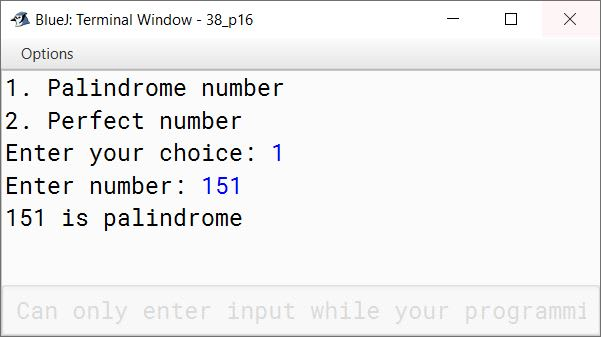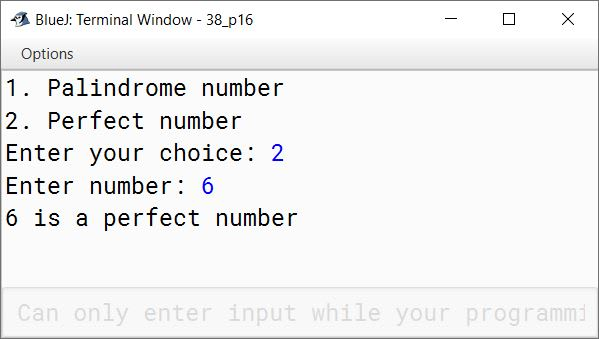#### Question 17

Write a menu driven program to accept a number from the user and check whether it is a Prime number or an Automorphic number.
(a) Prime number: (A number is said to be prime, if it is only divisible by 1 and itself)
Example: 3,5,7,11
(b) Automorphic number: (Automorphic number is the number which is contained in the last digit(s) of its square.)
Example: 25 is an Automorphic number as its square is 625 and 25 is present as the last two digits.

```import java.util.Scanner;

public class KboatPrimeAutomorphic
{
public static void main(String args[]) {
Scanner in = new Scanner(System.in);
System.out.println("1. Prime number");
System.out.println("2. Automorphic number");
int choice = in.nextInt();
System.out.print("Enter number: ");
int num = in.nextInt();

switch (choice) {
case 1:
int c = 0;
for (int i = 1; i <= num; i++) {
if (num % i == 0) {
c++;
}
}
if (c == 2)
System.out.println(num + " is Prime");
else
System.out.println(num + " is not Prime");
break;

case 2:
int numCopy = num;
int sq = num * num;
int d = 0;

/*
* Count the number of
* digits in num
*/
while(num > 0) {
d++;
num /= 10;
}

/*
* Extract the last d digits
* from square of num
*/
int ld = (int)(sq % Math.pow(10, d));

if (ld == numCopy)
System.out.println(numCopy + " is automorphic");
else
System.out.println(numCopy + " is not automorphic");
break;

default:
System.out.println("Incorrect Choice");
break;
}
}
}```
##### Output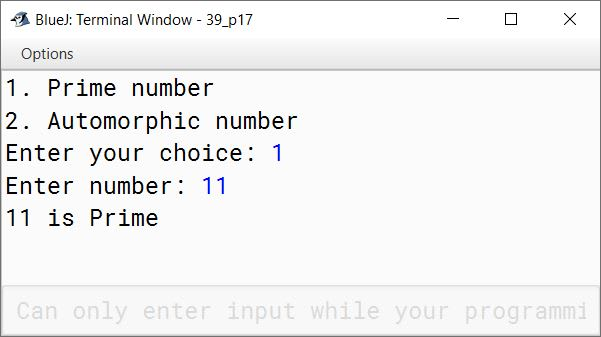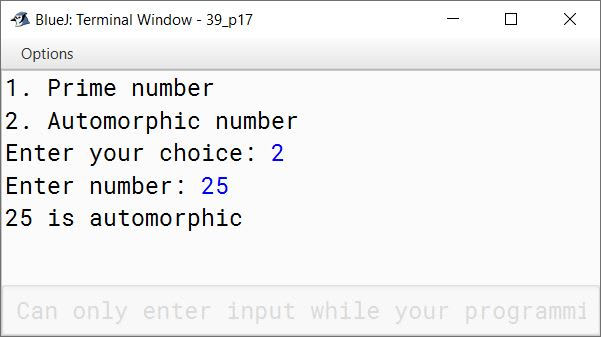#### Question 18

Write a menu driven program to perform the following tasks by using Switch case statement:
(a) To print the series:
0, 3, 8, 15, 24, ............ to n terms. (value of 'n' is to be an input by the user)
(b) To find the sum of the series:
S = (1/2) + (3/4) + (5/6) + (7/8) + ........... + (19/20)

```import java.util.Scanner;

{
public static void main(String args[]) {
Scanner in = new Scanner(System.in);
System.out.println("Type 1 to print series");
System.out.println("0, 3, 8, 15, 24,....to n terms");
System.out.println();
System.out.println("Type 2 to find sum of series");
System.out.println("(1/2) + (3/4) + (5/6) + (7/8) +....+ (19/20)");
System.out.println();
int choice = in.nextInt();

switch (choice) {
case 1:
System.out.print("Enter n: ");
int n = in.nextInt();
for (int i = 1; i <= n; i++)
System.out.print(((i * i) - 1) + " ");
System.out.println();
break;

case 2:
double sum = 0;
for (int i = 1; i <= 19; i = i + 2)
sum += i / (double)(i + 1);
System.out.println("Sum = " + sum);
break;

default:
System.out.println("Incorrect Choice");
break;
}
}
}```
##### Output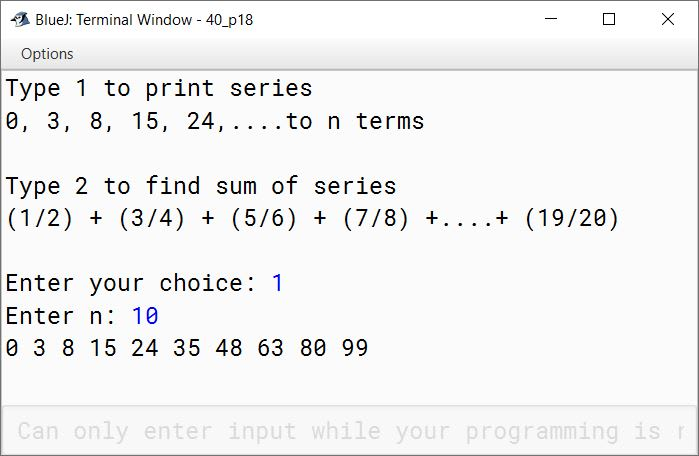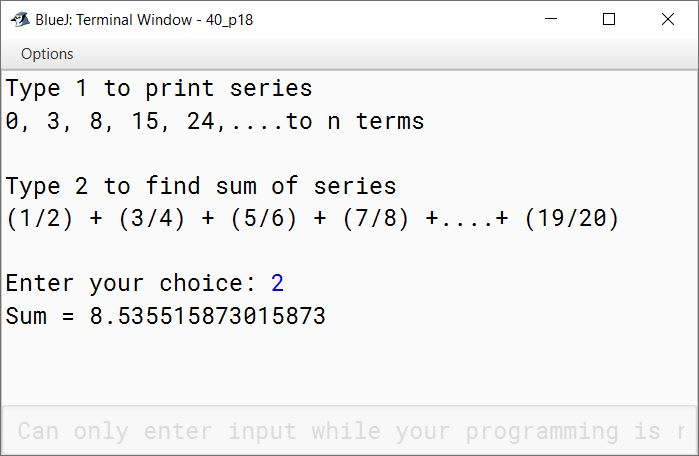#### Question 19

Using a switch statement, write a menu driven program to:
(a) Generate and display the first 10 terms of the Fibonacci series
0, 1, 1, 2, 3, 5
The first two Fibonacci numbers are 0 and 1, and each subsequent number is the sum of the previous two.
(b) Find the sum of the digits of an integer that is input.
Sample Input: 15390
Sample Output: Sum of the digits = 18
For an incorrect choice, an appropriate error message should be displayed.

```import java.util.Scanner;

public class KboatFibonacciNDigitSum
{
public static void main(String args[]) {
Scanner in = new Scanner(System.in);
System.out.println("1. Fibonacci Series");
System.out.println("2. Sum of digits");
int ch = in.nextInt();

switch (ch) {
case 1:
int a = 0, b = 1;
System.out.print(a + " " + b);
for (int i = 3; i <= 10; i++) {
int term = a + b;
System.out.print(" " + term);
a = b;
b = term;
}
break;

case 2:
System.out.print("Enter number: ");
int num = in.nextInt();
int sum = 0;
while (num != 0) {
sum += num % 10;
num /= 10;
}
System.out.println("Sum of Digits " + " = " + sum);
break;

default:
System.out.println("Incorrect choice");
break;
}
}
}```
##### Output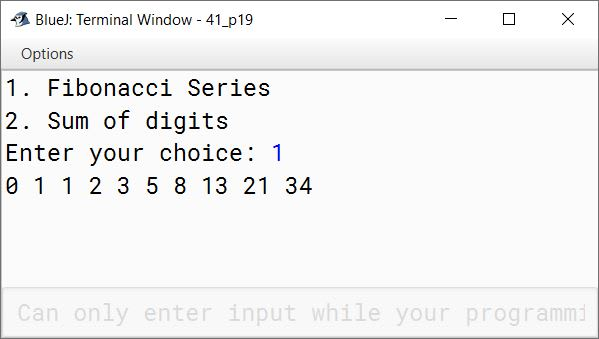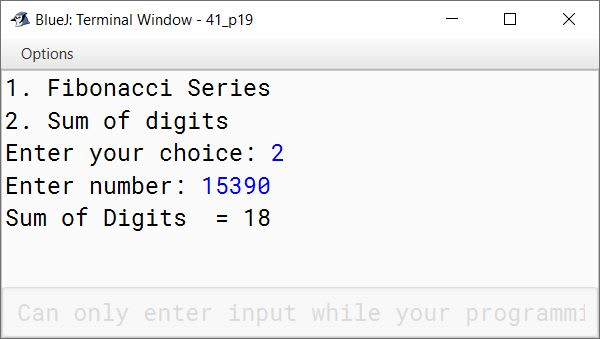#### Question 20

A special two-digit number is such that when the sum of its digits is added to the product of its digits, the result is equal to the original two-digit number.
Example: Consider the number 59.
Sum of digits = 5 + 9 = 14
Product of digits = 5 * 9 = 45
Sum of the sum of digits and product of digits = 14 + 45 = 59
Write a program to accept a two-digit number. Add the sum of its digits to the product of its digits. If the value is equal to the number input, then display the message "Special 2 - digit number" otherwise, display the message "Not a special two-digit number".

```import java.util.Scanner;

public class KboatSpecialNumber
{
public static void main(String args[]) {

Scanner in = new Scanner(System.in);

System.out.print("Enter a 2 digit number: ");
int orgNum = in.nextInt();

int num = orgNum;
int count = 0, digitSum = 0, digitProduct = 1;

while (num != 0) {
int digit = num % 10;
num /= 10;
digitSum += digit;
digitProduct *= digit;
count++;
}

if (count != 2)
System.out.println("Invalid input, please enter a 2-digit number");
else if ((digitSum + digitProduct) == orgNum)
System.out.println("Special 2-digit number");
else
System.out.println("Not a special 2-digit number");

}
}```
##### Output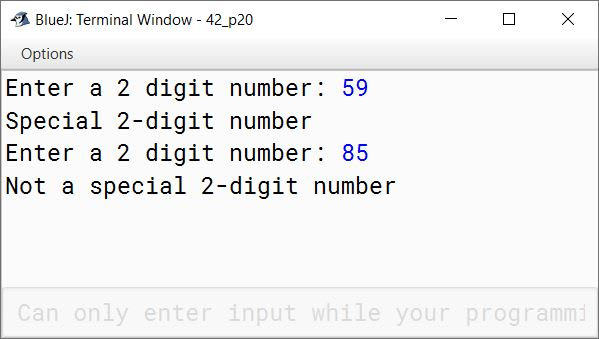#### Question 21

Using switch statement, write a menu driven program to:
(a) find and display all the factors of a number input by the user ( including 1 and the excluding the number itself).
Example: Sample Input : n = 15
Sample Output : 1, 3, 5
(b) find and display the factorial of a number input by the user (the factorial of a non-negative integer n, denoted by n!, is the product of all integers less than or equal to n.)
Example: Sample Input : n = 5
Sample Output : 5! = 1*2*3*4*5 = 120
For an incorrect choice, an appropriate error message should be displayed.

```import java.util.Scanner;

{
public static void main(String args[]) {
Scanner in = new Scanner(System.in);
System.out.println("1. Factors of number");
System.out.println("2. Factorial of number");
int choice = in.nextInt();
int num;

switch (choice) {
case 1:
System.out.print("Enter number: ");
num = in.nextInt();
for (int i = 1; i < num; i++) {
if (num % i == 0) {
System.out.print(i + " ");
}
}
System.out.println();
break;

case 2:
System.out.print("Enter number: ");
num = in.nextInt();
int f = 1;
for (int i = 1; i <= num; i++)
f *= i;
System.out.println("Factorial = " + f);
break;

default:
System.out.println("Incorrect Choice");
break;
}
}
}```
##### Output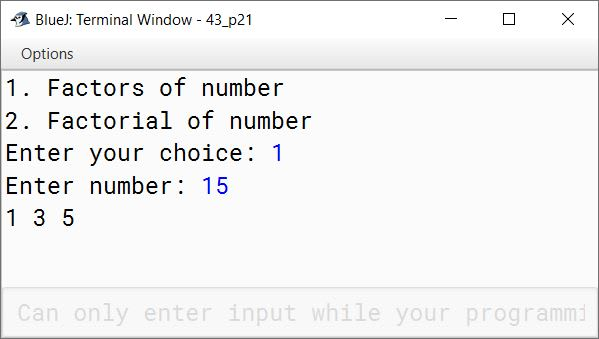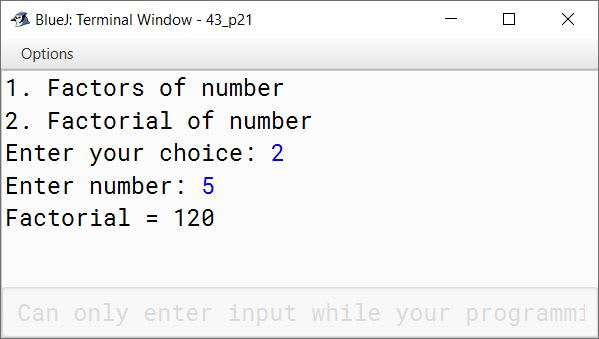#### Question 22

Write a program to input a number. Check and display whether it is a Niven number or not. (A number is said to be Niven which is divisible by the sum of its digits).
Example: Sample Input 126
Sum of its digits = 1 + 2 + 6 = 9 and 126 is divisible by 9.

```import java.util.Scanner;

public class KboatNivenNumber
{
public static void main(String args[]) {
Scanner in = new Scanner(System.in);
System.out.print("Enter number: ");
int num = in.nextInt();
int orgNum = num;

int digitSum = 0;

while (num != 0) {
int digit = num % 10;
num /= 10;
digitSum += digit;
}

/*
* digitSum != 0 check prevents
* division by zero error for the
* case when users gives the number
* 0 as input
*/
if (digitSum != 0 && orgNum % digitSum == 0)
System.out.println(orgNum + " is a Niven number");
else
System.out.println(orgNum + " is not a Niven number");
}
}```
##### Output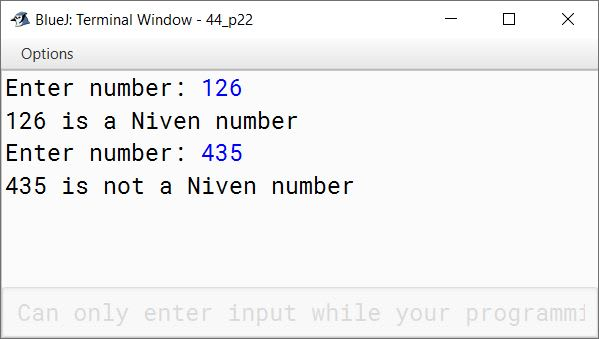#### Question 23

Write a program to accept a number and check whether it is a 'Spy Number' or not. (A number is spy if the sum of its digits equals the product of its digits.)
Example: Sample Input: 1124
Sum of the digits = 1 + 1 + 2 + 4 = 8
Product of the digits = 1*1*2*4 = 8

```import java.util.Scanner;

public class KboatSpyNumber
{
public static void main(String args[]) {

Scanner in = new Scanner(System.in);

System.out.print("Enter Number: ");
int num = in.nextInt();

int digit, sum = 0;
int orgNum = num;
int prod = 1;

while (num > 0) {
digit = num % 10;

sum += digit;
prod *= digit;
num /= 10;
}

if (sum == prod)
System.out.println(orgNum + " is Spy Number");
else
System.out.println(orgNum + " is not Spy Number");

}
}```
##### Output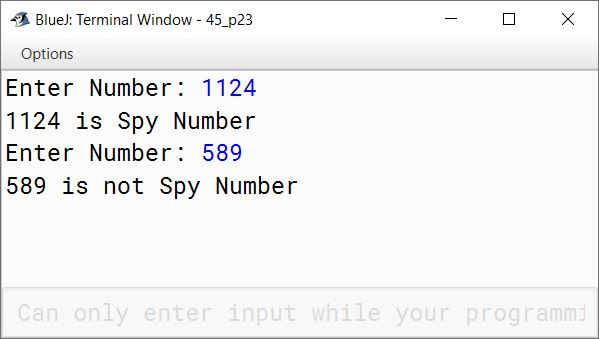#### Question 24

Using switch statement, write a menu driven program for the following:
(a) To find and display the sum of the series given below:
S = x1 - x2 + x3 - x4 + x5 - ............ - x20; where x = 2
(b) To display the series:
1, 11, 111, 1111, 11111
For an incorrect option, an appropriate error message should be displayed.

```import java.util.Scanner;

{
public static void main(String args[]) {
Scanner in = new Scanner(System.in);
System.out.println("1. Sum of the series");
System.out.println("2. Display series");
int choice = in.nextInt();

switch (choice) {
case 1:
int sum = 0;
for (int i = 1; i <= 20; i++) {
int term = (int)Math.pow(2, i);
if (i % 2 == 0)
sum -= term;
else
sum += term;
}
System.out.println("Sum=" + sum);
break;

case 2:
int term = 1;
for (int i = 1; i <= 5; i++) {
System.out.print(term + " ");
term = term * 10 + 1;
}
break;

default:
System.out.println("Incorrect Choice");
break;
}
}
}```
##### Output